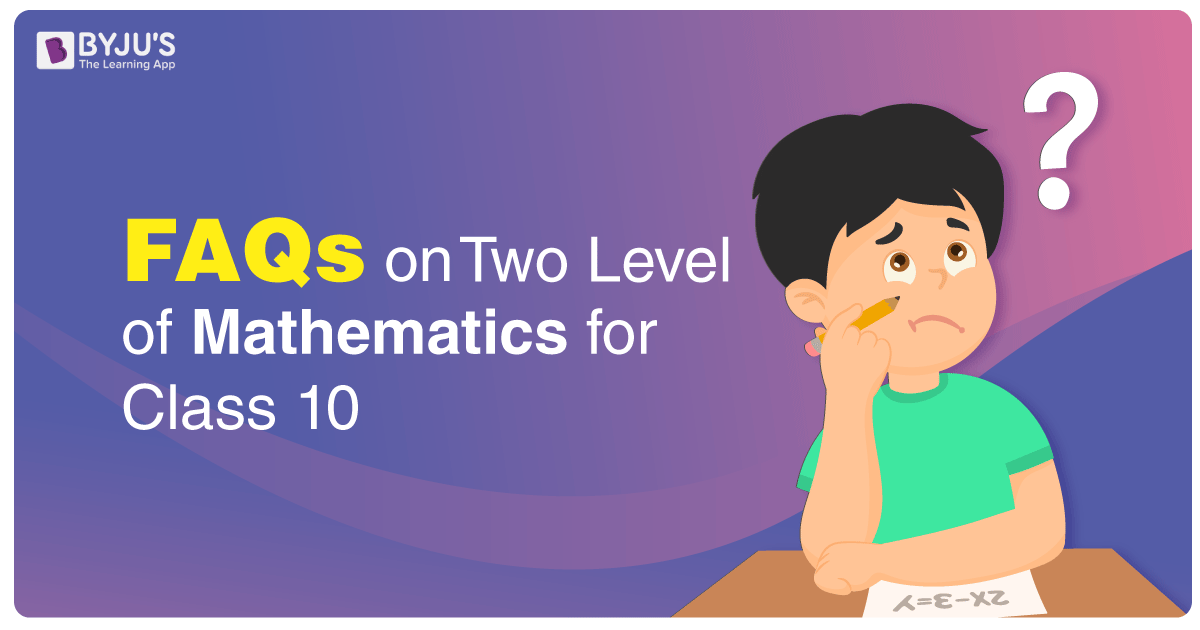# FAQs on Two Levels of Mathematics Exam 2020 Released by CBSEThe Central Board of Secondary Education (CBSE) has introduced two levels of Mathematics in Class 10. The board had already released the official notification regarding the same on 10th Jan 2019. Students have selected between the Basic and Standard Level of Maths paper at the time of submission of List of Candidates (LoC). Several doubts have arisen in students minds regarding the Basic and Standard Maths Paper, such as paper pattern, difficulty level of exam, etc. To clear all those doubts of Class 10 students, CBSE has released the FAQs on Two Levels of Mathematics Exam 2020.

Students can download the official pdf released by the board on two levels of Maths exam from the link provided above. However, for students’ convenience, we have provided the FAQs on this page as well. So, go through these FAQs and get a clear understanding of the Basic and Standard level Maths Class 10 exam.

Q1) What is the difference between the Standard Level and Basic Level of Mathematics in Class X Board examination to be held in 2020?

Ans: The existing Mathematics examination is the Standard Level Examination. Standard-Level and Basic-level Question papers shall be based on the same syllabus. However, the Standard-Level Mathematics assesses higher Mathematical abilities, compared to the Basic-Level. Accordingly, the difficulty level of Mathematics – ‘Basic’ is less than that of Mathematics-‘Standard’.

Q2) Are we increasing the burden on the child by introducing the concept of two Levels of Exams in Mathematics?

Ans: No, because the option to pick either Level is with the student. Further, the syllabus prescribed by CBSE will remain the same for both levels.

Q3) Will this lead to dilution of Mathematics syllabus/teaching?

Ans: No, the idea is to make the evaluation more student centric and reduce the pressure on the student who doesn’t want to pursue Mathematics after Class X.

Q4) Can a student opt for both Standard Level and Basic Level Exams?

Ans: No. There is only one option, either Mathematics-Standard or Mathematics-Basic.

Q5) To continue Mathematics as a subject in Class XI/XII and further, which Level Exam does a student need to pass?

Ans: Mathematics-Standard is to be passed at Class X, for taking up Mathematics at Sr. Secondary level.

Q6) If a student qualifies only the Mathematics-Basics in class X, can he/she opt for

Mathematics in class XI/XII?

Ans: No. If he/she intends to take Mathematics in class XI/XII, he/she has to pass Mathematics-Standard.

Q7) If a student has registered for the Basic Level Exam but wishes to appear for the Standard Level after the declaration of result, can he/she do so?

Ans: Yes. He/She can appear in the Standard Level at the Compartment examination, if he/she wishes to do so after passing the Basic Mathematics Examination.

Q8) How can I get the Sample Papers and Question Paper (Q.P) designs of Standard Level and Basic level Mathematics papers?

Ans: Sample papers and Blue Print based on the curriculum for 2019-20 will be made available on www.cbseacademic.nic.in., in due course of time.

The CBSE class 10 board exams are starting from 15th Feb 2020 and will continue till 20th Mar 2020. The Maths paper is scheduled on 12th March 2020. So, this is the time when solving the CBSE Class 10 Maths Sample Papers and Previous Years Class 10 Maths Question Papers will help in analysing the preparation level and thus, help in scoring good marks in the exam. So, use these resources and make your preparation more organised and effective.

All the Best for Board Exams!